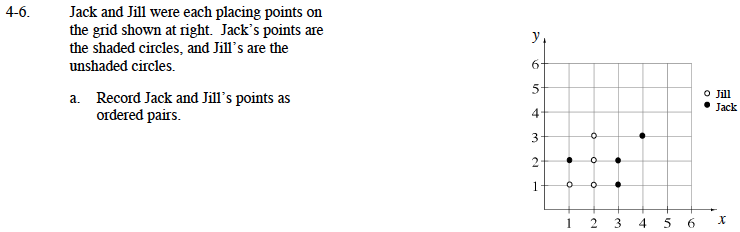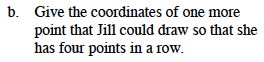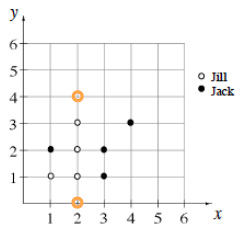### Home > MC1 > Chapter 4 > Lesson 4.1.1 > Problem4-6

4-6.Remember, in an ordered pair, the x coordinate (showing the placement along the x axis) is listed first and the y coordinate (showing the placement along the y axis) is listed second, as in (x, y).Can you spot the possible points? Here's a clue: the point should be at the two value on the x axis, so the ordered pair will look something like this (2, y).

Jill's ordered pairs are (1, 1), (2, 1), (2, 2), and (2, 3).
Can you name Jack's ordered pairs?The two possible points are now plotted. Can you record their ordered pairs?